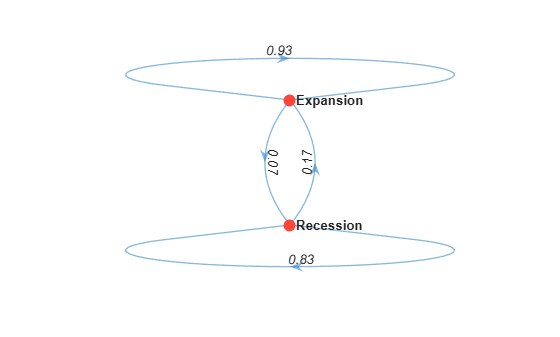# Work with State Transitions

This example shows how to work with transition data from an empirical array of state counts, and create a discrete-time Markov chain (`dtmc`) model characterizing state transitions. For each quarter in 1857–2022, the example categorizes the state of the US economy as experiencing a recession or expansion by using historical recession periods defined by the National Bureau of Economic Research (NBER). Then, the example forms the empirical transition matrix by counting the quarter-to-quarter transitions of the economic state.

### Load and Process Data

Load the historical, NBER-defined recession start and end dates.

`load Data_Recessions`

The `Recessions` variable in the workspace is a 34-by-2 matrix of serial date numbers. Rows of `Recessions` correspond to successive periods of recession, and columns 1 and 2 contain the start and end dates, respectively. For more details, enter `Description` at the command line.

Because MATLAB® `datetime` arrays enable you to work with date data efficiently, convert `Recessions` to a `datetime` array. Specify the date format `yyyy-MM-dd`. Visually compare the data types of the first few periods and the last few periods.

```NBERRec = datetime(Recessions,'ConvertFrom',"datenum",... "Format","yyyy-MM-dd"); disptbl = table(Recessions,NBERRec); head(disptbl,3)```
``` Recessions NBERRec ________________________ ______________________ 6.7842e+05 6.7897e+05 1857-06-15 1858-12-15 6.7964e+05 6.7988e+05 1860-10-15 1861-06-15 6.8128e+05 6.8226e+05 1865-04-15 1867-12-15 ```
`tail(disptbl,3)`
``` Recessions NBERRec ________________________ ______________________ 7.3092e+05 7.3117e+05 2001-03-15 2001-11-15 7.3339e+05 7.3394e+05 2007-12-15 2009-06-15 7.3784e+05 7.379e+05 2020-02-15 2020-04-15 ```

### Create Array of Visited States

Assume that the sampling period begins on `NBERRec(1,1)` = `1857-06-15` and ends on `2021-12-10`. Create a datetime array of all consecutive quarters in the interval `1857-06-15` through `2021-12-10`.

```timeline = NBERRec(1,1):calquarters(1):datetime("2021-12-10"); T = numel(timeline); % Sample size```

Identify which quarters occur during a recession.

```isrecession = @(x)any(isbetween(x,NBERRec(:,1),NBERRec(:,2))); idxrecession = arrayfun(isrecession,timeline);```

Create a MATLAB® timetable containing a variable that identifies the economic state of each quarter in the sampling period. Display the first few observations and the last few observations in the timetable.

```EconState = repmat("Expansion",T,1); % Preallocation EconState(idxrecession) = "Recession"; Tbl = timetable(EconState,'RowTimes',timeline); head(Tbl,3)```
``` Time EconState __________ ___________ 1857-06-15 "Recession" 1857-09-15 "Recession" 1857-12-15 "Recession" ```
`tail(Tbl,3)`
``` Time EconState __________ ___________ 2021-03-15 "Expansion" 2021-06-15 "Expansion" 2021-09-15 "Expansion" ```

`Tbl` is a data set of the observed, quarterly economic states, from which transitions can be counted.

### Count State Transitions

Consider creating a 2-by-2 matrix containing the number of:

• Transitions from a recession to an expansion

• Transitions from an expansion to a recession

• Self-transitions of recessions

• Self-transitions of expansions

Row $\mathit{j}$ and column $\mathit{j}$ of the matrix correspond to the same economic state, and element $\left(\mathit{j},\mathit{k}\right)$ contains the number of transitions from state $\mathit{j}$ to state $\mathit{k}$.

First, assign indices to members of the state space.

```[idxstate,states] = grp2idx(Tbl.EconState); states```
```states = 2x1 cell {'Recession'} {'Expansion'} ```
```numstates = numel(states); P = zeros(numstates); % Preallocate transition matrix```

The state indices `idxstate` correspond to states (rows and columns) of the transition matrix, and `states` defines the indices. `P(1,1)` is the number of self-transitions of recessions, `P(1,2)` is the number of transitions from a recession to an expansion, and so on. Therefore, to count the transition types in the data efficiently, for every transition $\mathit{t}$ to $\mathit{t}+1$, tally the observed transition by indexing into the transition matrix using state indices at times $\mathit{t}$ (row) and $\mathit{t}+1$ (column).

```for t = 1:(T - 1) P(idxstate(t),idxstate(t+1)) = P(idxstate(t),idxstate(t+1)) + 1; end array2table(P,'VariableNames',states,'RowNames',states)```
```ans=2×2 table Recession Expansion _________ _________ Recession 171 34 Expansion 33 419 ```

`P` is the matrix of observed transitions. `P(1,2)` = `34` means that the US economy transitioned from a recession to an expansion `34` times between `1857-06-15` and `2021-12-10`.

### Create Discrete-Time Markov Chain

Create a discrete-time Markov chain model characterized by the transition matrix `P`.

```mc = dtmc(P,'StateNames',states); array2table(mc.P,'VariableNames',states,'RowNames',states)```
```ans=2×2 table Recession Expansion _________ _________ Recession 0.83415 0.16585 Expansion 0.073009 0.92699 ```

`mc` is a `dtmc` object. `dtmc` normalizes `P` to create the right-stochastic transition matrix `mc.P` (each row sums to 1). The element `mc.P(1,2)` = `0.1659` means that the empirical probability of transitioning to an expansion at time $\mathit{t}$ + 1, given that the US economy is in a recession at time $\mathit{t}$, is 0.1659.

Plot a directed graph of the Markov chain.

`graphplot(mc,'LabelEdges',true)`The Markov chain suggests that the current economic state tends to persist, from quarter to quarter.